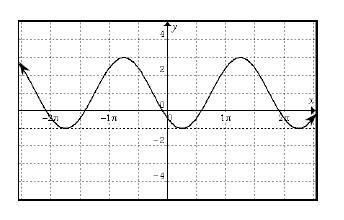### Home > PC > Chapter 4 > Lesson 4.3.1 > Problem4-127

4-127.

Using the smallest possible horizontal shift, find an equation for the graph below in terms of:1. $\text{sin }x$

Draw in the mid-line and max and min lines. Then determine the amplitude, period, and shifts.

2. $\text{cos }x$

Use the eTool below to visualize this problem.
Click on the link to the right to view the full version of the eTool: PCT 4-127 eTool